# HashMap：从源码分析到面试题

IdentityServer4 (3) 授权码模式(Authorization Code)

## HashMap简介

HashMap是实现map接口的一个重要实现类，在我们无论是日常还是面试，以及工作中都是一个经常用到角色。它的结构如下：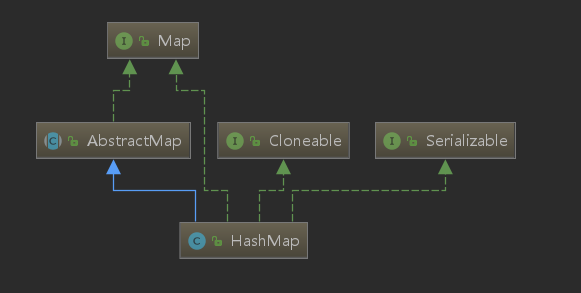### 哈希表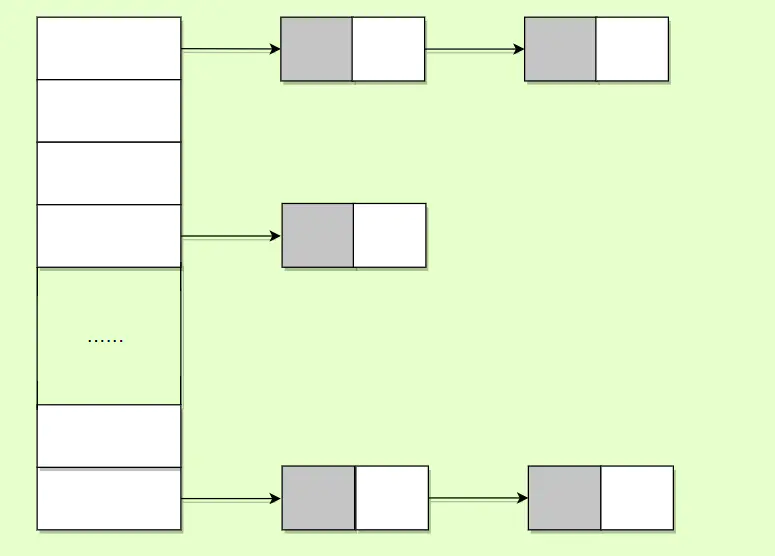## 源码解析

### 基本属性

1、我们的默认的初始化的hashmap的容量，如果没有指定的话，就是我们的默认，1<<4就是16。

``````    static final int DEFAULT_INITIAL_CAPACITY = 1 << 4; // aka 16
``````

2、我们的hashmap最大容量，2的30次方。

``````    static final int MAXIMUM_CAPACITY = 1 << 30;
``````

3、默认的装载因子，0.75。有什么用呢？比如我们的容量现在是16，16*0.75=12，也就是说，当我们的实际容量到了12的时候，那么就会触发扩容机制，进行扩容！

``````    static final float DEFAULT_LOAD_FACTOR = 0.75f;
``````

4、我们知道哈希表是由数组和链表组成的，每一个位置都可以说是一个哈希桶。我们的哈希桶默认是链表，但是在JDK1.8之后我们的哈希桶中当有TREEIFY_THRESHOLD个节点的时候，也就是下面默认的8，我们桶中的链表会被转换为红黑树的结构。

``````    static final int TREEIFY_THRESHOLD = 8;
``````

5、与上面相同，不过不同的是，会将红黑树转换成链表。

``````    static final int UNTREEIFY_THRESHOLD = 6;
``````

6、当哈希桶的结构转换成树之前，还会有一次判断，只有键值对大于64才会转换！也就是我们下面定义的最小容量，这是为了避免哈希表建立初期多个键值对恰巧都在一个哈希桶上面，而导致了没必要的转换。

``````    static final int MIN_TREEIFY_CAPACITY = 64;
``````

7、内部结构静态内部类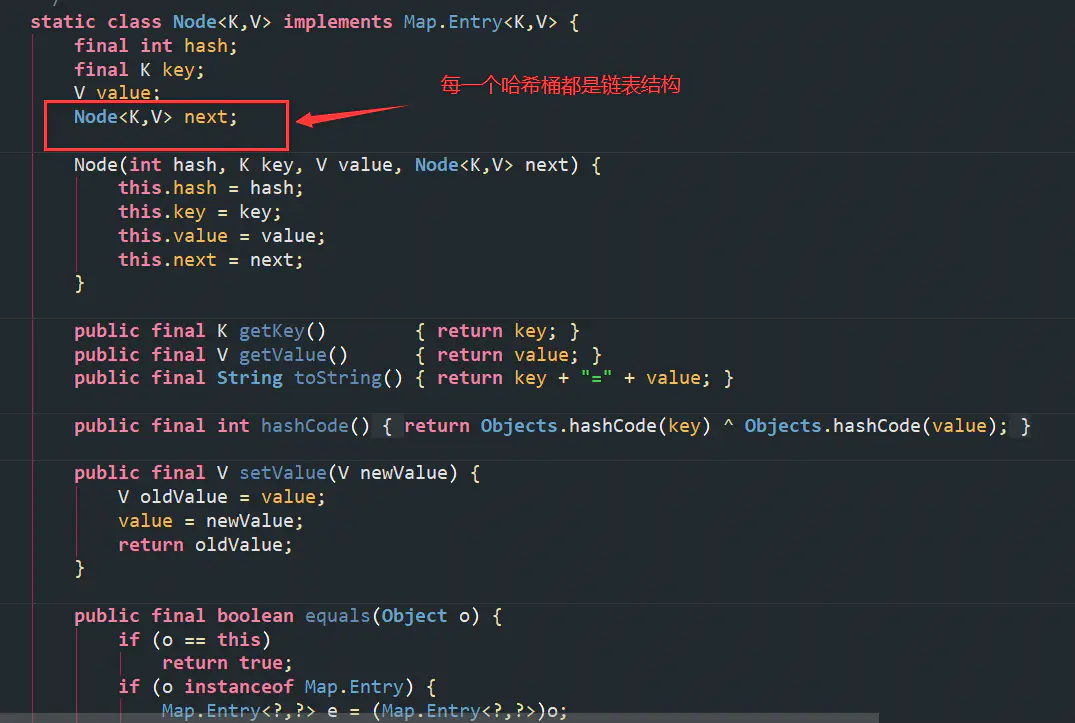8、其他成员变量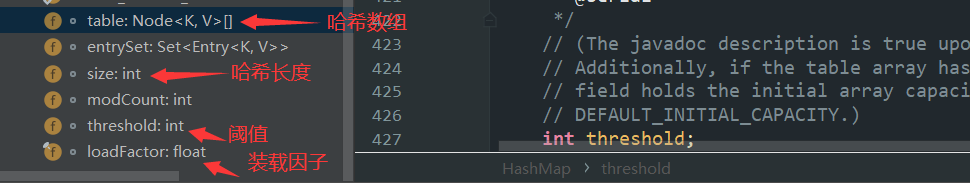### 构造方法

hashmap的构造方法有四个，不过我们重点介绍其中的一个，因为这一个理解了，其他的也不成问题。

``````//initialCapacity：初始大小
public HashMap(int initialCapacity, float loadFactor) {
if (initialCapacity < 0)
throw new IllegalArgumentException("Illegal initial capacity: " +
initialCapacity);
if (initialCapacity > MAXIMUM_CAPACITY)
initialCapacity = MAXIMUM_CAPACITY;
throw new IllegalArgumentException("Illegal load factor: " +
this.threshold = tableSizeFor(initialCapacity);
}
``````

1. 首先是边界处理，如果初始大小小于0，抛异常。如果大于最大，那也只能赋予我们默认的最大值！如果装载因子小于0或者不是数字的话，抛异常！
2. 然后就是进行我们的赋值，装载因子赋值，还有就是调用我们的`tableSizeFor`来返回一个大于等于initialCapacity的2次幂。
``````    static final int tableSizeFor(int cap) {
int n = cap - 1;
n |= n >>> 1;
n |= n >>> 2;
n |= n >>> 4;
n |= n >>> 8;
n |= n >>> 16;
return (n < 0) ? 1 : (n >= MAXIMUM_CAPACITY) ? MAXIMUM_CAPACITY : n + 1;
}
``````

``````this.threshold = tableSizeFor(initialCapacity) * this.loadFactor;
``````

### 核心方法

#### put方法

``````    public V put(K key, V value) {
return putVal(hash(key), key, value, false, true);
}
``````

``````    static final int hash(Object key) {
int h;
return (key == null) ? 0 : (h = key.hashCode()) ^ (h >>> 16);
}
``````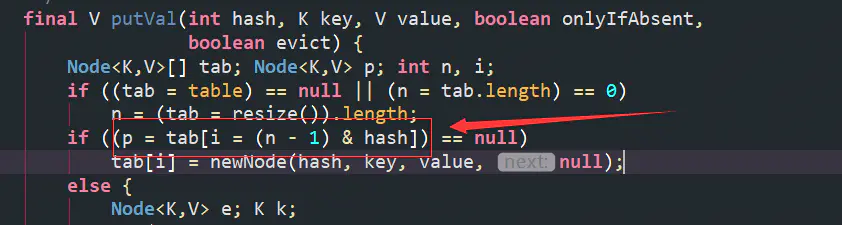``````比如我们的长度转换为2进制为 1000 0000 ，进行-1操作后就是 0111 1111

1000 0000
&
0101 1011 = 0000 0000

0111 1111
&
0101 1011 = 0101 1011

``````

length = 8 ，（length-1） = 7 ， 转换二进制为111；

``````    0000 0100 1011 0011 1101 1111 1110 0001

&运算

0000 0000 0000 0000 0000 0000 0000 0111

=   0000 0000 0000 0000 0000 0000 0000 0001 （就是十进制1，所以下标为1）
``````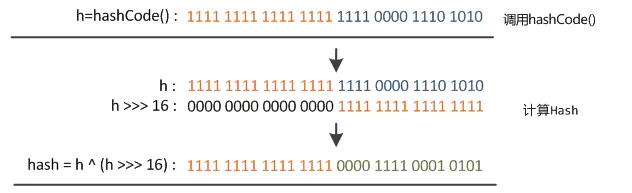• 当length=8时 下标运算结果取决于哈希值的低三位

• 当length=16时 下标运算结果取决于哈希值的低四位

消息队列和事件循环（Event Loop）

• 当length=32时 下标运算结果取决于哈希值的低五位

• 当length=2的N次方， 下标运算结果取决于哈希值的低N位。

``````    final V putVal(int hash, K key, V value, boolean onlyIfAbsent,
boolean evict) {
Node<K,V>[] tab; Node<K,V> p; int n, i;
//当我们的table为空的时候调用resize()进行扩容初始化
if ((tab = table) == null || (n = tab.length) == 0)
n = (tab = resize()).length;
// 没有发生碰撞，初始化我们的第一个节点
if ((p = tab[i = (n - 1) & hash]) == null)
tab[i] = newNode(hash, key, value, null);
//发生碰撞
else {
Node<K,V> e; K k;
if (p.hash == hash &&
((k = p.key) == key || (key != null && key.equals(k))))
//hashcode和key相等，记录下原先的值
e = p;
//如果这个时候我们的哈希桶已经是红黑树结构，那么调用树的插入函数
else if (p instanceof TreeNode)
e = ((TreeNode<K,V>)p).putTreeVal(this, tab, hash, key, value);
else {
//链表结构，同时我们的hashcode不相等
//找到与key相等的节点，更新value，退出循环
//如果没有找到与key相等的节点，在链表尾部插入，如果插入后节点数量大于
//我们变成红黑树的阈值，那么进行转换成红黑树
for (int binCount = 0; ; ++binCount) {
if ((e = p.next) == null) {
p.next = newNode(hash, key, value, null);
//达到临界值转换成红黑树
if (binCount >= TREEIFY_THRESHOLD - 1) // -1 for 1st
treeifyBin(tab, hash);
break;
}
if (e.hash == hash &&
((k = e.key) == key || (key != null && key.equals(k))))
break;
p = e;
}
}
//新值覆盖旧值，返回旧值
if (e != null) { // existing mapping for key
V oldValue = e.value;
if (!onlyIfAbsent || oldValue == null)
e.value = value;
afterNodeAccess(e);
return oldValue;
}
}
++modCount;
//键值对达到阈值，进行扩容
if (++size > threshold)
resize();
afterNodeInsertion(evict);
return null;
}
``````

#### resize()方法

``````   final Node<K,V>[] resize() {
//原table数组赋值
Node<K,V>[] oldTab = table;
//如果原数组为null，那么原数组长度为0
int oldCap = (oldTab == null) ? 0 : oldTab.length;
//赋值阈值
int oldThr = threshold;
//newCap 新数组长度
//newThr 下次扩容的阈值
int newCap, newThr = 0;
// 1. 如果原数组长度大于0
if (oldCap > 0) {
//如果大于最大长度1 << 30 = 1073741824，那么阈值赋值为Integer.MAX_VALUE后直接返回
if (oldCap >= MAXIMUM_CAPACITY) {
threshold = Integer.MAX_VALUE;
return oldTab;
}
// 2. 如果原数组长度的2倍小于最大长度，并且原数组长度大于默认长度16，那么新阈值为原阈值的2倍
else if ((newCap = oldCap << 1) < MAXIMUM_CAPACITY &&
oldCap >= DEFAULT_INITIAL_CAPACITY)
newThr = oldThr << 1; // double threshold
}
// 3. 如果原数组长度等于0，但原阈值大于0，那么新的数组长度赋值为原阈值大小
else if (oldThr > 0) // initial capacity was placed in threshold
newCap = oldThr;
else {               // zero initial threshold signifies using defaults
// 4. 如果原数组长度为0，阈值为0，那么新数组长度，新阈值都初始化为默认值
newCap = DEFAULT_INITIAL_CAPACITY;
}
// 5.如果新的阈值等于0
if (newThr == 0) {
//计算临时阈值
float ft = (float)newCap * loadFactor;
//新数组长度小于最大长度，临时阈值也小于最大长度，新阈值为临时阈值，否则是Integer.MAX_VALUE
newThr = (newCap < MAXIMUM_CAPACITY && ft < (float)MAXIMUM_CAPACITY ?
(int)ft : Integer.MAX_VALUE);
}
//计算出来的新阈值赋值给对象的阈值
threshold = newThr;
@SuppressWarnings({"rawtypes","unchecked"})
//用新计算的数组长度新建一个Node数组，并赋值给对象的table
Node<K,V>[] newTab = (Node<K,V>[])new Node[newCap];
table = newTab;
//后面是copy数组和链表数据逻辑
if (oldTab != null) {
for (int j = 0; j < oldCap; ++j) {
Node<K,V> e;
if ((e = oldTab[j]) != null) {
oldTab[j] = null;
if (e.next == null)
newTab[e.hash & (newCap - 1)] = e;
else if (e instanceof TreeNode)
((TreeNode<K,V>)e).split(this, newTab, j, oldCap);
else { // preserve order
Node<K,V> loHead = null, loTail = null;
Node<K,V> hiHead = null, hiTail = null;
Node<K,V> next;
do {
next = e.next;
if ((e.hash & oldCap) == 0) {
if (loTail == null)
else
loTail.next = e;
loTail = e;
}
else {
if (hiTail == null)
else
hiTail.next = e;
hiTail = e;
}
} while ((e = next) != null);
if (loTail != null) {
loTail.next = null;
}
if (hiTail != null) {
hiTail.next = null;
}
}
}
}
}
return newTab;
}
``````

``````//①
Map<String, String> map = new HashMap<>();
map.put("1", "1");
//②
Map<String, String> map1 = new HashMap<>(2);
map1.put("2", "2");
//③
Map<String, String> map2 = new HashMap<>(2, 0.5f);
map2.put("3", "3");
``````
• ① 没有设置initialCapacity，也没有设置负载因子，第一次put的时候会触发扩容。第一次的时候，数组长度为默认值16，阈值为160.75=12，走的`代码4`逻辑，等到数组长度超过阈值12后，触发第二次扩容，此时table数组，和threshold都不为0，即oldTab、oldCap、oldThr都不为0，先走`代码1`，如果oldCap长度的2倍没有超过最大容量，并且oldCap 长度大于等于 默认容量16，那么下次扩容的阈值 变为oldThr大小的两倍即 12 2 = 24，newThr = 24，newCap=32
• ② 设置了initialCapacity，没有设置负载因子，此时hashMap使用默认负载因子0.75，本实例设置的初始容量为2，通过计算阈值为2，第一次put的时候由于还没初始化table数组，因此触发第一次扩容。此时oldCap为0，oldThr为2，走`代码3`，确定这次扩容的新数组大小为2，此时还没有确定newThr 下次扩容的大小，于是进入`代码5` 确定newThr为 2 0.75 = 1.5 取整 1 ，及下次扩容阈值为1。当数组已有元素大于阈值及1时，触发第二次扩容，此时oldCap为1，oldThr为1，走`代码1`newCap = oldCap << 1 结果为 4 小于最大容量， 但oldCap 小于hashMap默认大小16，结果为false，跳出判断，此时由于newThr等于0，进入`代码5`，确定newThr为 4 0.75 = 3，下次扩容阈值为3
• ③ 设置了initialCapacity=2，负载因子为0.5，通过tableSizeFor计算阈值为2，第一次put的时候，进行扩容，此时oldCap为2，oldThr为2，进入`代码1`，同实例②，newCap = oldCap << 1 结果为 4 小于最大容量， 但oldCap 小于hashMap默认大小16，结果为false，跳出判断，进入`代码5`，确定newThr为 4 * 0.5 = 2,下次扩容阈值为2

#### get()方法

``````    public V get(Object key) {
Node<K,V> e;
return (e = getNode(hash(key), key)) == null ? null : e.value;
}
``````

``````    final Node<K,V> getNode(int hash, Object key) {
Node<K,V>[] tab; Node<K,V> first, e; int n; K k;
//如果计算出来的哈希值是在哈希表上
if ((tab = table) != null && (n = tab.length) > 0 &&
(first = tab[(n - 1) & hash]) != null) {
//如果刚好在哈希桶的第一个节点上，返回
if (first.hash == hash && // always check first node
((k = first.key) == key || (key != null && key.equals(k))))
return first;
//如果不在第一个节点，遍历节点
if ((e = first.next) != null) {
//如果这个时候我们的哈希桶已经是树形结构了，调用树形查找
if (first instanceof TreeNode)
return ((TreeNode<K,V>)first).getTreeNode(hash, key);
//对我们的链表进行遍历查找元素
do {
if (e.hash == hash &&
((k = e.key) == key || (key != null && key.equals(k))))
return e;
} while ((e = e.next) != null);
}
}
//如果没有找到的话，返回null
return null;
}
``````

#### remove方法

``````    public V remove(Object key) {
Node<K,V> e;
return (e = removeNode(hash(key), key, null, false, true)) == null ?
null : e.value;
}
``````

``````    final Node<K,V> removeNode(int hash, Object key, Object value,
boolean matchValue, boolean movable) {
Node<K,V>[] tab; Node<K,V> p; int n, index;
//我们的哈希桶不为空，同时要映射的哈希值也在
if ((tab = table) != null && (n = tab.length) > 0 &&
(p = tab[index = (n - 1) & hash]) != null) {
Node<K,V> node = null, e; K k; V v;
//刚好我们的哈希桶首位就是要删除的，记录下来
if (p.hash == hash &&
((k = p.key) == key || (key != null && key.equals(k))))
node = p;
//如果不是，进行遍历查找
else if ((e = p.next) != null) {
//如果是红黑树结构的话，调用树的查找方法
if (p instanceof TreeNode)
node = ((TreeNode<K,V>)p).getTreeNode(hash, key);
else {
//对链表进行查找key
do {
if (e.hash == hash &&
((k = e.key) == key ||
(key != null && key.equals(k)))) {
node = e;
break;
}
p = e;
} while ((e = e.next) != null);
}
}
//找到了之后就去删除，分红黑树，桶的首位，链表中，
if (node != null && (!matchValue || (v = node.value) == value ||
(value != null && value.equals(v)))) {
//红黑树，调用树删除节点的方法
if (node instanceof TreeNode)
((TreeNode<K,V>)node).removeTreeNode(this, tab, movable);
//在桶首位，直接将下一个节点赋值给首位
else if (node == p)
tab[index] = node.next;
//在链表中，将下一个结点赋值给前一个节点的下一个节点，删除自我
else
p.next = node.next;
++modCount;
--size;
afterNodeRemoval(node);
return node;
}
}
return null;
}
``````

## 总结

1. 扩容是一个特别耗性能的操作，所以当我们在使用 HashMap，正确估算 map 的大小，初始化的时候给一个大致的数值，避免 map 进行频繁的扩容。

3. HashMap 是非线程安全的，不要在并发的情况下使用 HashMap，建议使用 ConcurrentHashMap！

## 面试题分析

1、为什么要将转换成树形结构的阈值设置为8呢？为什么不将转换成链表结构的阈值也设置为8呢？

1. 当初始阈值为8时，链表的长度达到8的概率变的很小，如果再大概率减小的并不明显

2. 树结构查找的时间复杂度是O(log(n))，而链表的时间复杂度是O(n)，当阈值为8时，long8 = 3，相比链表更快，但树结构比链表占用的空间更多，所以这是一种时间和空间的平衡

2、HashMap 为什么不用平衡树，而用红黑树？

• 红黑树也是一种平衡树，但不是严格平衡，平衡树是左右子树高度差不超过1，红黑树可以是2倍

• 红黑树在插入、删除的时候旋转的概率比平衡树低很多，效率比平衡树高

3、HashMap在并发下会产生什么问题？有什么替代方案?

HashMap并发下产生问题：由于在发生hash冲突，插入链表的时候，多线程会造成环链，再get的时候变成死循环，Map.size()不准确，数据丢失。

• HashTable: 通过synchronized来修饰，效率低，多线程put的时候，只能有一个线程成功，其他线程都处于阻塞状态
• ConcurrentHashMap：
1.7 采用锁分段技术提高并发访问率
1.8 数据依旧是分段存储，但锁采用了synchronized

4、HashMap中的key可以是任何对象或数据类型吗？

• 可以是null，但不能是可变对象，如果是可变对象，对象中的属性改变，则对象的HashCode也相应改变，导致下次无法查找到已存在Map中的数据

• 如果要可变对象当着键，必须保证其HashCode在成员属性改变的时候保持不变

5、为什么不直接将key作为哈希值而是与高16位做异或运算？

6、关于更多的面试题

## HashMap与HashTable有什么不同？

Hashtable是个过时的集合类，不建议在新代码中使用，不需要线程安全的场合可以用HashMap替换，需要线程安全的场合可以用ConcurrentHashMap替换或者Collections的synchronizedMap方法使HashMap具有线程安全的能力。

Iterator遍历数组顺序 索引从小到大 索引从大到小

## 参考资料

4.Java容器-HashMap详解

HashMap源码注解 之 静态工具方法hash()、tableSizeFor()（四）

HashMap中hash(Object key)原理，为什么(hashcode >>> 16)。

HashMap：从源码分析到面试题

synchronized 锁的原理